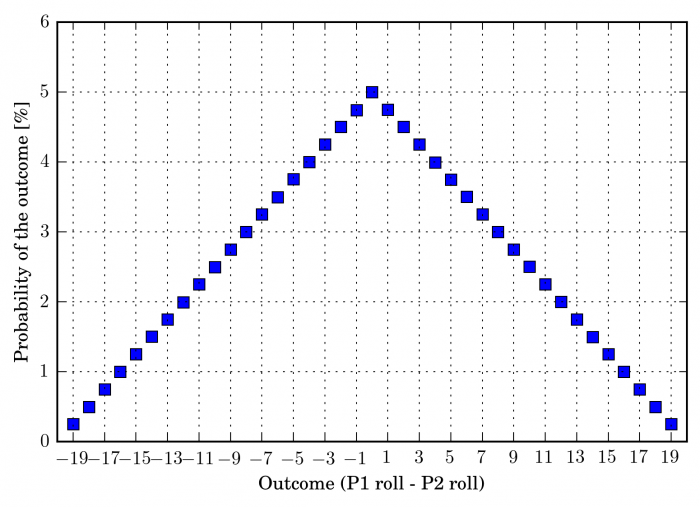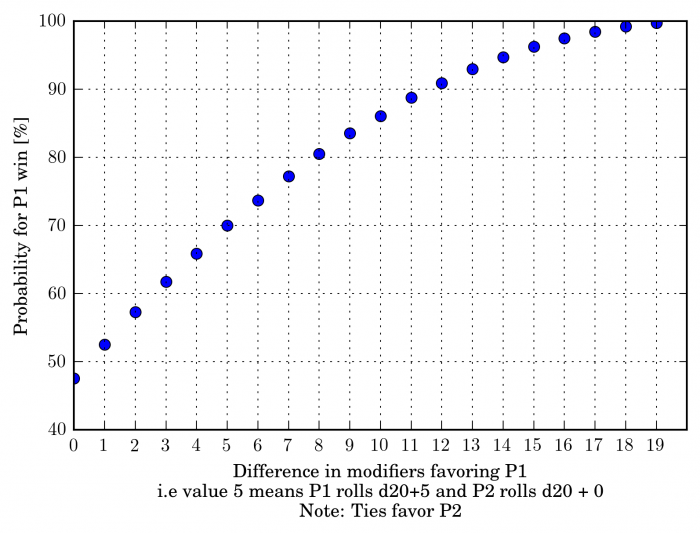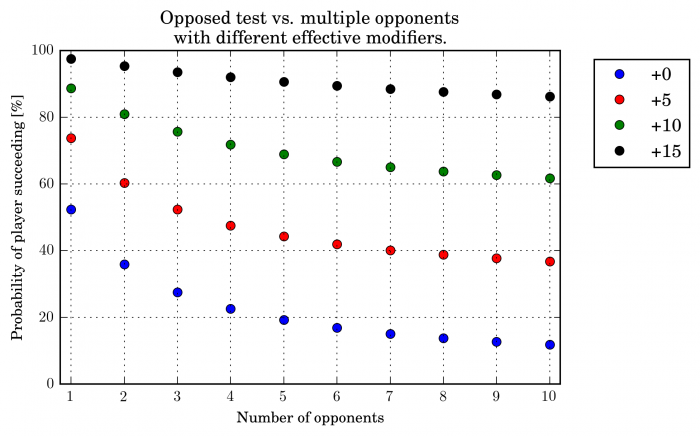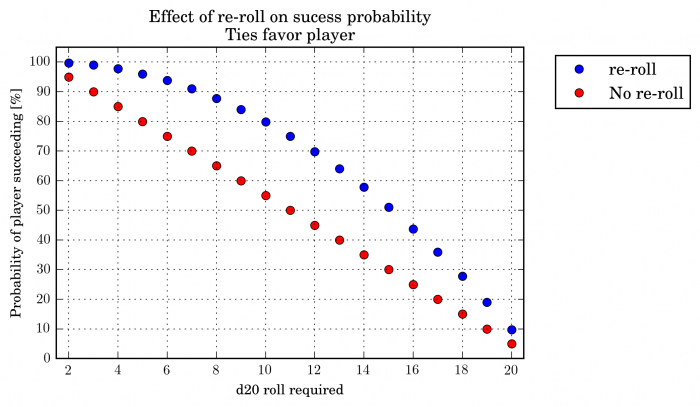# Dice math, part 1 of N

Most RPGs feature at least some mechanics with uncertainty and from both the designer and player perspective, it is important to know what you get out of them. Often their properties are intuitively clear, but I thought it’d be fun to go through them in more detail than usually happens in a gaming session. Even though pieces like this might be available somewhere, I wanted to go through the math and simulations myself. This is part 1 of N of series where I’ll go through the properties of different mechanics. I’ll start with a staple: A linear random number generator (RNG, usually a die / dice). The most popular example of this is probably D&D and it’s spawn (eg pathfinder).

Linear RNG is very simple and it is almost too trivial to go over, but it has a few interesting cases. The system is straightforward, a die roll + (modifiers) compared to some value. Each value of the die is equally likely i.e. the probability for each is 1/N where N is the number of die faces. For the rest of this post, I’ll be using d20 system as an example, but it is straightforward to generalize. For a d20 die the expected value is $$EV(d20) = \sum_{i=1}^{20} \frac{i}{20} = 10.5$$

The probability of succeeding given a target number of A – assuming success on a roll equal to or greater than the target number –  is then $$P(success) = \frac{20 - (A-M-1)}{20} = \frac{21 + M - A}{20},$$

where M is the player’s modifier to the roll. For example, player rolls d20+5 vs threshold of 15 yields P(success) = 55%. Also note that if the modifier M is larger than the threshold A – 1, success is automatic.

An opposed test is a little more complex, but not overwhelmingly so. First, let’s go over the notation. The test is usually just a comparison of who gets the highest score. Occasionally, the actual difference . Heads up situation is very simple and – as you’d expect – 50-50. But it’s noteworthy that extreme differences are less likely than smaller ones. Consider an example; Intuitively, there is only one way to have a difference of -19, i.e. one roll of 1 and other being 20, whereas for -18 there are two possibilities: 2 and 20 and 1 and 19. This means that we should observe difference of -18 twice as often as difference of -19. A figure should illustrate this nicely.Addition of modifiers does not change the situation much, although it should be noted that it makes some results impossible. For example, if the difference in modifiers favors player 1 by +5 – i.e. P1 rolls d20+5 and P2 d20 – then P1 can’t lose by more than -14 (P1 rolls 1 and P2 20, difference is 1+5-20 = -14), i.e. probability for getting a difference of -15 is 0. Also, consider the situations where the bonus difference is +18 and +17. In the former case, there is only one possibility for the other player to win, that P1 rolls 20 and P2 rolls 1. In the latter case, P1 can win three ways: 20 vs 1, 19 vs 1 or 20 vs 2, meaning that the value of a modifier point is different depending on the original situation. To illustrate the point, consider the following table and figure that gives winning probability as a function of difference in bonuses for values +0 to +19.So a linear RNG is simple to understand from both design and player perspective especially if target numbers are static. To summarize the properties of the main mechanic:

1. Very easy to grasp intuitively. A +1 adds 5 percentage points (for d20) to the tests success probability vs a static threshold. This changes a bit for opposed tests.
2. RNG vs static bonus -balance. It might be hard to formulate numbers such that the amount of randomness is on the expected level, especially if character’s abilities change significantly during the the game. For example, for starting characters in D&D 3/Pathfinder, the RNG completely dominates most checks but the situation is completely reversed when the characters reach higher levels. Of course the effective modifiers – meaning relation between thresholds and the player’s bonuses – can be set in a such way that the ratio remains constant.
3. “Off the RNG”, i.e. one character always wins (or loses) in a test. For example in D&D 3e (and it’s descendants), it’s not unusual to have bonuses diverge by more than 20 even in relatively low levels. This means that a specialist always wins against a non-specialist. This could be more of a feature than a bug, but still something to keep in mind especially from the design perspective.
4. Granular RNG leads to large modifier numbers, which slows down resolution time (adding large numbers is slower than adding small numbers) but manageable numbers lead to less granularity: d20 has 20 distinct outcomes.
5. Things are linear: A 2nd level fighter (ceteris paribus) has a 5% advantage over a 1st level fighter at hitting things. Any exponential growth scheme probably just fucks many things up or makes numbers unwieldy.

Finally, let’s go over some “special” or nice to know cases. First multiple opposition: Eg. a player tries to sneak past multiple guards and each of them tries to hear the player. Often this is modeled as an opposed skill test, but instead of heads on, the player has to beat the best result of the opposition. So the criterion for success becomes (d20 + modifiers – max(opposition skill test) > 0). It’s easier to assume identical opposition and do a quick and dirty Monte Carlo -simulation. The results are exactly what you’d expect. As the number of opponents grows, the probability of one of them rolling 20 increases until the player is essentially facing a static threshold (20 + bonus of the opponent). This however happens only for very many opponents. The probability of the player winning is plotted in the next figure for a few different effective modifier, i.e. the difference between players modifier and the opponents modifier. E.g. the player’s bonus is +7, oppositions bonus is +4, and the resulting effective modifier is +3 for the player.Most of the interesting things happen at the very start. Going from 1 to 3 opponents already drops the success chance quite a lot. Also note that the effect is much less important for high effective modifiers. For example +15 modifier favoring the player means that if the player rolls 5 or higher (assuming ties favor the player) wins automatically no matter what the rolls of the opposition are (again, assuming no special rules beyond simple comparison). Also note that the effect is relatively mild for test where the player has already a large advantage.

This is already nearing a thousand words, so I’m quickly going to comment on effect of re-rolls. First, an unconditional re-roll, i.e. a situation where two dice are rolled and the higher of the two chosen, increases the average result from 10.5 to roughly 13.8 meaning that it’s in game mechanics terms worth about the same as a +3 static bonus. This naturally doesn’t apply if the first roll cannot be chosen. First, consider the case where the re-roll can be applied after knowing if the result was successful. For example, against threshold 10 (again, ties are wins, no modifiers, so just d2o must be 10 or higher), the win probability goes from 55% to about 80% with the ability to re-roll on failure. Similarly for dc 15 the probability goes from 30% to 51%. Clearly, the ability to re-roll after knowing the result of the first test is very powerful. The following figure should illustrate the change.Finally, the case of choosing to re-roll before knowing if the first roll succeeded or not (choosing to re-roll forfeits the previous roll). This is much tougher since it requires estimation of the difficulty of the task at hand which is often not available. However, it is very easy to count the probability of rolling higher (or lower) than the initial roll. For example, the probability for rolling higher than 12 is 40%. The rest is just “game mastery”.

In next part, I’ll cover the dicepool mechanic as used in Shadowrun or World of Darkness.